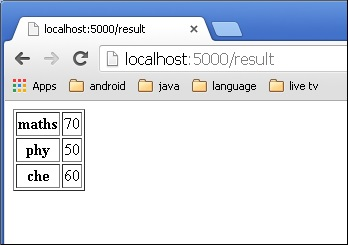# Flask – Templates

Advertisements

It is possible to return the output of a function bound to a certain URL in the form of HTML. For instance, in the following script, hello() function will render ‘Hello World’ with <h1> tag attached to it.

```from flask import Flask
app = Flask(__name__)

@app.route('/')
def index():
return '<html><body><h1>Hello World</h1></body></html>'

if __name__ == '__main__':
app.run(debug = True)
```

However, generating HTML content from Python code is cumbersome, especially when variable data and Python language elements like conditionals or loops need to be put. This would require frequent escaping from HTML.

This is where one can take advantage of Jinja2 template engine, on which Flask is based. Instead of returning hardcode HTML from the function, a HTML file can be rendered by the render_template() function.

```from flask import Flask
app = Flask(__name__)

@app.route('/')
def index():
return render_template(‘hello.html’)

if __name__ == '__main__':
app.run(debug = True)
```

Flask will try to find the HTML file in the templates folder, in the same folder in which this script is present.

• Application folder
• Hello.py
• templates
• hello.html

The term ‘web templating system’ refers to designing an HTML script in which the variable data can be inserted dynamically. A web template system comprises of a template engine, some kind of data source and a template processor.

Flask uses jinja2 template engine. A web template contains HTML syntax interspersed placeholders for variables and expressions (in these case Python expressions) which are replaced values when the template is rendered.

The following code is saved as hello.html in the templates folder.

```<!doctype html>
<html>
<body>

<h1>Hello {{ name }}!</h1>

</body>
</html>
```

Next, run the following script from Python shell.

```from flask import Flask, render_template
app = Flask(__name__)

@app.route('/hello/<user>')
def hello_name(user):
return render_template('hello.html', name = user)

if __name__ == '__main__':
app.run(debug = True)
```

As the development server starts running, open the browser and enter URL as − http://localhost:5000/hello/mvl

The variable part of URL is inserted at {{ name }} place holder.The jinja2 template engine uses the following delimiters for escaping from HTML.

• {% ... %} for Statements
• {{ ... }} for Expressions to print to the template output
• {# ... #} for Comments not included in the template output
• # ... ## for Line Statements

In the following example, use of conditional statement in the template is demonstrated. The URL rule to the hello() function accepts the integer parameter. It is passed to the hello.html template. Inside it, the value of number received (marks) is compared (greater or less than 50) and accordingly HTML is conditionally rendered.

The Python Script is as follows −

```from flask import Flask, render_template
app = Flask(__name__)

@app.route('/hello/<int:score>')
def hello_name(score):
return render_template('hello.html', marks = score)

if __name__ == '__main__':
app.run(debug = True)
```

HTML template script of hello.html is as follows −

```<!doctype html>
<html>
<body>
{% if marks>50 %}
<h1> Your result is pass!</h1>
{% else %}
<h1>Your result is fail</h1>
{% endif %}
</body>
</html>
```

Note that the conditional statements if-else and endif are enclosed in delimiter {%..%}.

Run the Python script and visit URL http://localhost/hello/60 and then http://localhost/hello/30 to see the output of HTML changing conditionally.

The Python loop constructs can also be employed inside the template. In the following script, the result() function sends a dictionary object to template results.html when URL http://localhost:5000/result is opened in the browser.

The Template part of result.html employs a for loop to render key and value pairs of dictionary object result{} as cells of an HTML table.

Run the following code from Python shell.

```from flask import Flask, render_template
app = Flask(__name__)

@app.route('/result')
def result():
dict = {'phy':50,'che':60,'maths':70}
return render_template('result.html', result = dict)

if __name__ == '__main__':
app.run(debug = True)
```

Save the following HTML script as result.html in the templates folder.

```<!doctype html>
<html>
<body>
<table border = 1>
{% for key, value in result.items() %}
<tr>
<th> {{ key }} </th>
<td> {{ value }} </td>
</tr>
{% endfor %}
</table>
</body>
</html>
```

Here, again the Python statements corresponding to the For loop are enclosed in {%..%} whereas, the expressions key and value are put inside {{ }}.

After the development starts running, open http://localhost:5000/result in the browser to get the following output.Advertisements